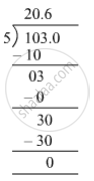SSC (English Medium) Class 8Maharashtra State Board
Share

# Write the Following Rational Number in Decimal Form. − 103 5 - SSC (English Medium) Class 8 - Mathematics

ConceptConcept for Decimal Representation of Rational Numbers

#### Question

Write the following rational number in decimal form.

(-103)/5

#### Solution

The given number is -103/5 .therefore 103/5 = 20.6

"The decimal form of"  -103/5  "is"  -20.6.

Is there an error in this question or solution?

#### APPEARS IN

Balbharati Solution for Balbharati Class 8 Mathematics (2019 to Current)
Chapter 1: Rational and Irrational numbers
Practice Set 1.3 | Q: 4 | Page no. 4
Solution Write the Following Rational Number in Decimal Form. − 103 5 Concept: Concept for Decimal Representation of Rational Numbers.
S